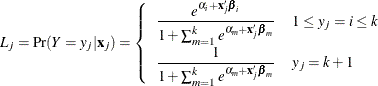Determining Observations for Likelihood Contributions

If you use events/trials MODEL statement syntax, split each observation into two observations. One has response value 1 with a frequency equal to the frequency of the original observation (which is 1 if the FREQ statement is not used) times the value of the events variable. The other observation has response value 2 and a frequency equal to the frequency of the original observation times the value of (trials–events). These two observations will have the same explanatory variable values and the same FREQ and WEIGHT values as the original observation.

For either single-trial or events/trials syntax, let j index all observations. In other words, for single-trial syntax, j indexes the actual observations. And, for events/trials syntax, j indexes the observations after splitting (as described in the preceding paragraph). If your data set has 30 observations and you use single-trial syntax, j has values from 1 to 30; if you use events/trials syntax, j has values from 1 to 60.

Suppose the response variable in a cumulative response model can take on the ordered values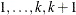, where k is an integer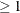. The likelihood for the jth observation with ordered response value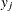and explanatory variables vector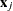is given by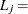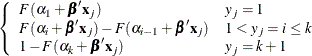where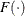is the logistic, normal, or extreme-value distribution function,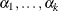are ordered intercept parameters, andis the common slope parameter vector.

For the generalized logit model, letting the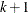st level be the reference level, the interceptsare unordered and the slope vector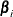varies with each logit. The likelihood for the jth observation with response valueand explanatory variables vectoris given by